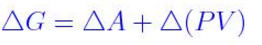# Gibbs Free Energy Formula

## Willard Gibbs defined a function known as Gibbs energy (G), to calculate the changes in entropy and enthalpy values. The maximum work done is the amount of energy produced, given by the decrease in the thermodynamic property called Gibbs free energy.

Gibbs Free energy formula is independent of temperature and pressure and as given below.

G = H – TS

Gibbs free energy has an extensive property and a function with the single value. Since H = U + PV by definition, Gibbs Free Energy Formula can be stated as

##G = (U + PV) – TS = A + PV

For a finite system, change in Gibbs free energy is written asWhen the pressure and temperature are constant, the equation becomes

Example 1

Determine the standard Gibbs free energy change for the creation of methane from carbon and hydrogen at 298 K. Given ΔH = -74.9KJ/mol and ΔS = -80.7J/K.mol

Solution

ΔH = -74.9KJ/mole

ΔS = -80.7J/K.mol

We have the equation

ΔG = ΔH – T ΔS

Substitute the above values in this equation

ΔG = -74.9 KJ/mol – (298 K) (-80.7J/K.mol) (1KJ/1000J)

ΔG = -50.9KJ/mole

Example 2

Determine the standard free energy change for the following reaction at 25oC.

N2 + 3H2 —> 2NH3

Given ΔH and S are -91.8KJ and -198.0J/K

Solution

We have an equation

G = H – TS

Substitute the above values in this equation

ΔG = -91.8KJ – (298 K) (-0.1980KJ/K)

ΔG = -32.8KJ

To refer more problems and questions on Gibbs Free energy Formula, please visit Byju’s.com